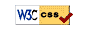# UM Math 115 On-Line Homework W23

## Course Selector

Read the hints and tips before taking a test. Select your section, and click "go to homework."

 Select Section Then Click section #
Note:
• Assignment due dates are shown in the homework.

## Hints and Tips

1. Start early, when each set opens.
2. Do the example homework set, Chap0 or homework0, or similar. It's easy & will help you with the homework system.
3. Don't submit partial answers. You have six submissions per problem.
4. Work the problems out by hand before entering them in the system. You can get a hardcopy of a problem set from the problem listing page of a problem set.
1. Carefully parenthesize. Type `cos(2pi)`, `e^(-ln(3))`, or `1/(1+x)`, not `cos 2pi`, `e^-ln3` or `1/1+x`. Note that `sin2x` is read as `sin(2)*x`, which is probably not what you want. Enter `sin(2x)` instead.
2. The square root is `sqrt(x)` or `x^(1/2)`.
7. Watch your case: `X` is different from `x`, `Sin` is different from `sin`, and `Pi` is different from `pi`. You want `pi`.UM Math 115 On-Line HW